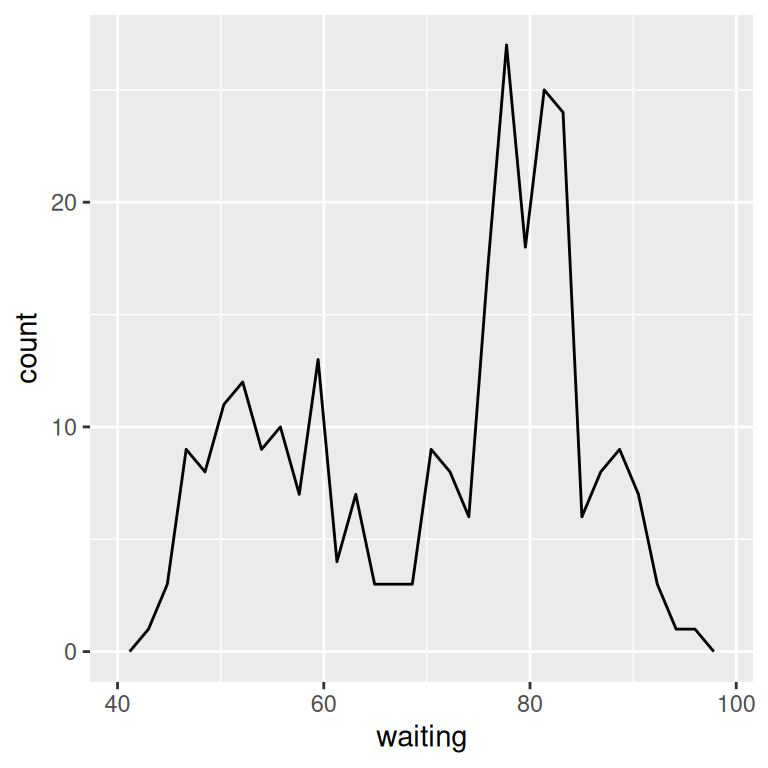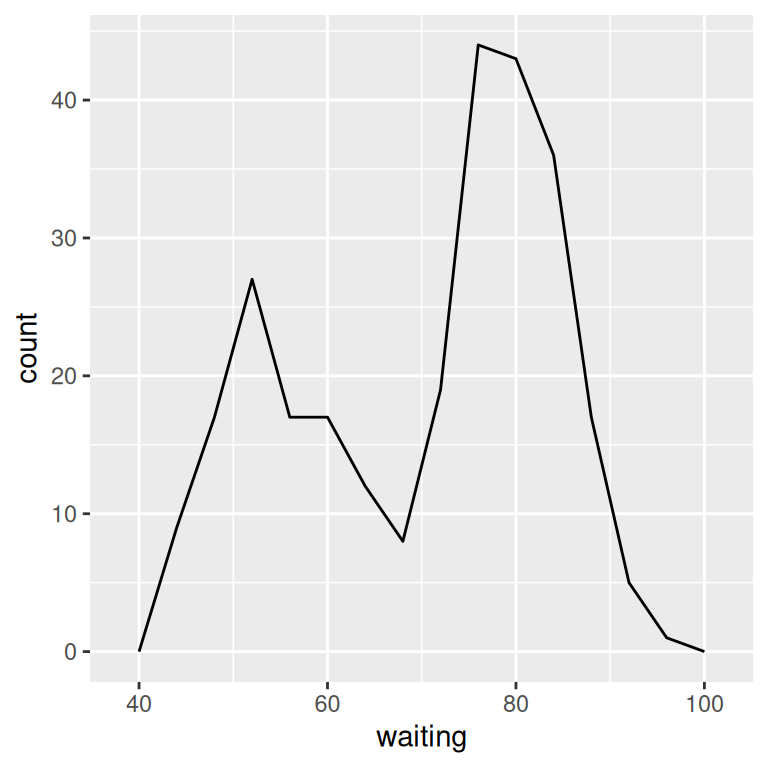## 6.5 Making a Frequency Polygon

### 6.5.1 Problem

You want to make a frequency polygon.

### 6.5.2 Solution

Use geom_`freqpoly()` (Figure 6.15):

``````ggplot(faithful, aes(x=waiting)) +
geom_freqpoly()``````

### 6.5.3 Discussion

A frequency polygon appears similar to a kernel density estimate curve, but it shows the same information as a histogram. That is, like a histogram, it shows what is in the data, whereas a kernel density estimate is just that – an estimate – and requires you to pick some value for the bandwidth.

Like with a histogram, you can control the bin width for the frequency polygon (Figure 6.15, right):

``````ggplot(faithful, aes(x = waiting)) +
geom_freqpoly(binwidth = 4)``````Figure 6.15: A frequency polygon (left); With wider bins (right)

Or, instead of setting the width of each bin directly, you can divide the x range into a particular number of bins:

``````# Divide the x-axis range into 15 bins
binsize <- diff(range(faithful\$waiting))/15

ggplot(faithful, aes(x = waiting)) +
geom_freqpoly(binwidth = binsize)``````